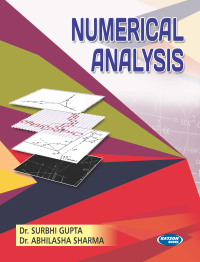•## Numerical Analysis

In Stock
ISBN 978-93-5014-541-8 Dr. Surbhi Gupta & Dr. Abhilasha Sharma S.K. Kataria & Sons 2014 Print In Stock
Rs 265.00Rs 239.00

#### CONTENTS

• High Speed Computations and Errors • Solution of Algebraic and Transcendental Equations • Solution of Linear Simultaneous Equations and Matrix Inversion Method and Eigen Values • Finite Differences • Interpolution with Equal Intervals • Interpolation with Unequal Intervals • Central Difference Interpolation Formulae • Numerical Differentiation and Integration • Numerical Integration • Solution of Differential Equations Numerical Solution of Ordinary Differential Equations • Curve Fitting by Method of Least Square and Cubic Spline • Linear Programming Problem • Statistical Computation • Model Test Papers • Examination Papers • Index.

• Author : Dr. Surbhi Gupta & Dr. Abhilasha Sharma
• Publisher : S.K. Kataria & Sons
• Publishing Year : 2014